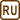## Mean Free Path of Hydrogen

The mean free path of hydrogen molecule under normal conditions is 130 nm. What is the hydrogen molecule diameter and what is its mean free path at normal temperature but at the pressure of 133.3·10−4 Pa?

• #### Hint 1

The Assignment mentions normal conditions. What does it mean?

• #### Hint 2 – Molecule Diameter

To determine unknown diameter d of hydrogen molecule, use a relationship for mean free path $$\bar{\lambda}$$.

• #### Hint 3 – Mean Free Path Under Lower Pressure

To determine mean free path $$\bar{\lambda}_2$$ under lower pressure p2 use the fact that distance is inversely proportional to pressure.

• #### Given Values

 $$\bar{\lambda}_{1}=130\,\mathrm{nm}=1.3\cdot{10^{-7}}\,\mathrm{m}$$ mean free path of hydrogen molecule under normal conditions p2 = 133.3·10−4 Pa lower pressure

Table values:

 T1 = 273.15 K normal temperature p1 = 101 325 Pa normal pressure k = 1.38 ·10−23 JK−1 Boltzmann constant
• #### Analysis

Mean free path indicates an average distance travelled by a molecule between two collisions. It is determined by a relation from statistical physics which states that it is directly proportional to thermodynamic temperature and inversely proportional to pressure and molecule diameter.

We can now determine unknown molecule diameter from the above mentioned relation.

When determinig mean free path under lower pressure we use the fact that this distance is inversely proportional to pressure.

• #### Solution

Mean free path $$\bar{\lambda}_1$$ is given by

$\bar{\lambda}_1=\frac{kT_1}{p_1 \pi d^{2} \sqrt{2}},$

where T1 is thermodynamic temperature under normal conditions, p1 normal pressure, d molecule diameter and k Boltzmann constant.

We can determine unknown diameter d of the molecule from this formula:

$d^{2}=\frac{kT_{1}}{p_{1} \pi \bar{\lambda_{1}} \sqrt{2}}$ $d=\sqrt{\frac{kT_{1}}{p_{1} \pi \bar{\lambda_{1}} \sqrt{2}}}.$

During the calculation of mean free path $$\bar{\lambda}_2$$ under lower pressure p2 we use the fact that this distance is inversely proportional to pressure. Provided that temperature remains constant (which is this case), the relationship must apply between the values of mean free path and related pressure

$\frac{\bar{\lambda}_{1}}{\bar{\lambda}_{2}}=\frac{p_{2}}{p_{1}}.$

After adjusting this relation we obtain a final equation for mean free path $$\bar{\lambda}_{2}$$ under lower pressure p2:

$\bar{\lambda}_{2}=\frac{p_{1}\bar{\lambda}_{1}}{p_{2}}.$
• #### Číselné dosazení

$d=\sqrt{\frac{kT_{1}}{p_{1} \pi \bar{\lambda_{1}} \sqrt{2}}}=\sqrt{\frac{1.38\cdot{10^{-23}}\cdot{273,.5}}{101\,325\cdot \pi\cdot{1.3}\cdot{10^{-7}}\cdot \sqrt{2}}}\,\mathrm{m}$ $d\dot{=}2.54\cdot{10^{-10}}\,\mathrm{m}=0.254\,\mathrm{nm}$ $\bar{\lambda}_{2}=\frac{p_{1}\bar{\lambda}_{1}}{p_{2}}=\frac{101\,325\cdot{1.3}\cdot{10^{-7}}}{133.3\cdot{10^{-4}}}\, \mathrm{m}\dot{=}0.99\, \mathrm{m}$